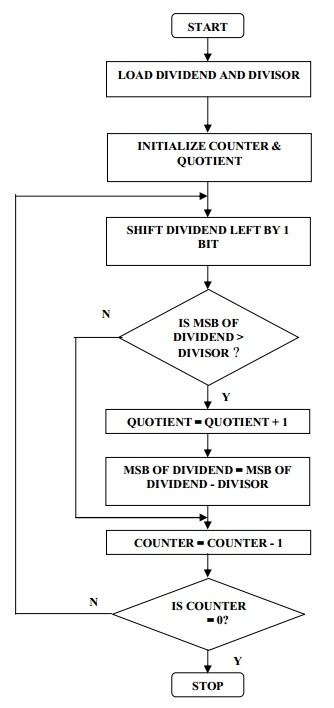# Program to Divide two 8 Bit numbers in 8085 Microprocessor

Here we will see 8085 program. This program will divide two 8-bit numbers using 8085 microprocessor.

Problem Statement

Write an 8085 Assembly language program to divide two 8-bit numbers and store the result at locations 8020H and 8021H.

Discussion

The 8085 has no division operation. To get the result of division, we should use the repetitive subtraction method.

By using this program, we will get the quotient and the remainder. 8020H will hold the quotient, and 8021H will hold remainder.

We are saving the data at location 8000H and 8001H. The result is storing at location 8050H and 8051H.

## Input

The Dividend: 0EH

The Divisor 04H

The Quotient will be 3, and remainder will be 2

## Flow Diagram## Program

HEX Codes
Labels
Mnemonics
F000
21, 0E, 00
START
LXI H,0CH
Load 8-bit dividend in HL register pair
F003
06, 04

MVI B,04H
Load divisor in B to perform num1 / num2
F005
0E, 08

MVI C, 08
Initialize the counter
F007
29
UP
Shifting left by 1 bit HL = HL + HL
F008
7C

MOV A, H
F009
90

SUB B
perform A = A – B
F00A
DA, 0F, F0

JC DOWN
If MSB<divisor then shift to left
F00D
67

MOV H,A
If MSB>divisor, store the current value of A in H
F00E
2C

INR L
Tracking quotient
F00F
0D
DOWN
DCR C
Decrement the counter
F010
C2, 07, F0

JNZ UP
If not exhausted then go again
F013
22, 20, 80

SHLD 8020
Store the result at 8020 H
F016
76

HLT
Stop